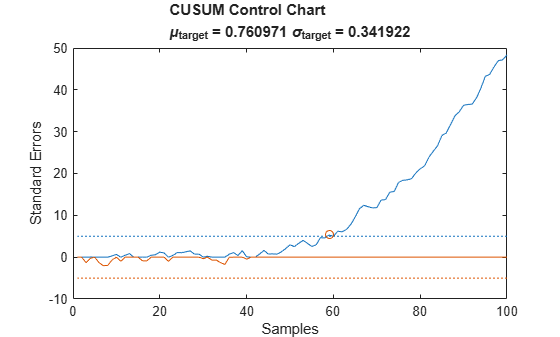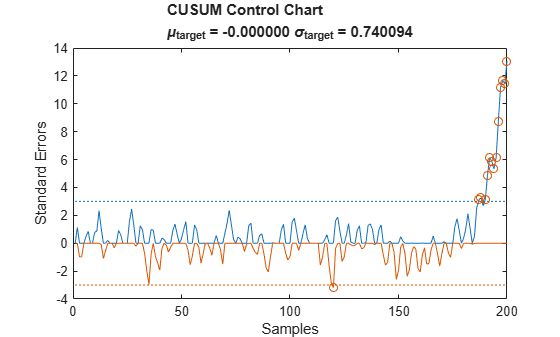# cusum

Detect small changes in mean using cumulative sum

## Syntax

``[iupper,ilower] = cusum(x)``
``[iupper,ilower] = cusum(x,climit,mshift,tmean,tdev)``
``[iupper,ilower] = cusum(___,'all')``
``````[iupper,ilower,uppersum,lowersum] = cusum(___)``````
``cusum(___)``

## Description

example

````[iupper,ilower] = cusum(x)` returns the first index of the upper and lower cumulative sums of `x` that have drifted beyond five standard deviations above and below a target mean. The minimum detectable mean shift is set to one standard deviation. The target mean and standard deviations are estimated from the first 25 samples of `x`.```

example

````[iupper,ilower] = cusum(x,climit,mshift,tmean,tdev)` specifies `climit`, the number of standard deviations that the upper and lower cumulative sums are allowed to drift from the mean. It also specifies the minimum detectable mean shift, the target mean, and the target standard deviation.```
````[iupper,ilower] = cusum(___,'all')` returns all the indices at which the upper and lower cumulative sums exceed the control limit.```

example

``````[iupper,ilower,uppersum,lowersum] = cusum(___)``` also returns the upper and lower cumulative sums.```
````cusum(___)` with no output arguments plots the upper and lower cumulative sums normalized to one standard deviation above and below the target mean.```

## Examples

collapse all

Generate and plot a 100-sample random signal with a linear trend. Reset the random number generator for reproducible results.

```rng('default') rnds = rand(1,100); trnd = linspace(0,1,100); fnc = rnds + trnd; plot(fnc)```Apply `cusum` to the function using the default values of the input arguments.

`cusum(fnc)`Compute the mean and standard deviation of the first 25 samples. Apply `cusum` using these numbers as the target mean and the target standard deviation. Highlight the point where the cumulative sum drifts more than five standard deviations beyond the target mean. Set the minimum detectable mean shift to one standard deviation.

```mfnc = mean(fnc(1:25)); sfnc = std(fnc(1:25)); cusum(fnc,5,1,mfnc,sfnc)```Repeat the calculation using a negative linear trend.

```nnc = rnds - trnd; cusum(nnc)```Generate a signal resembling motion about an axle that becomes unstable due to wear. Add white Gaussian noise of variance 1/9. Reset the random number generator for reproducible results.

```rng default sz = 200; dr = airy(2,linspace(-14.9371,1.2,sz)); rd = dr + sin(2*pi*(1:sz)/5) + randn(1,sz)/3;```

Plot the growing background drift and the resulting signal.

```plot(dr) hold on plot(rd,'.-') hold off```Find the mean and standard deviation if the drift is not present and there is no noise. Plot the ideal noiseless signal and its stable background.

```id = 0.3*sin(2*pi*(1:sz)/20); st = id + sin(2*pi*(1:sz)/5); mf = mean(st)```
```mf = -3.8212e-16 ```
`sf = std(st)`
```sf = 0.7401 ```
```plot(id) hold on plot(st,'.-') hold off```Use the CUSUM control chart to pinpoint the onset of instability. Assume that the system becomes unstable when the signal is three standard deviations beyond its ideal behavior. Specify a minimum detectable shift of one standard deviation.

`cusum(rd,3,1,mf,sf)`Make the violation criterion more strict by increasing the minimum detectable shift. Return all instances of unwanted drift.

`cusum(rd,3,1.2,mf,sf,'all')`Every hole in golf has an associated "par" that indicates the expected number of strokes needed to sink the ball. Skilled players usually complete each hole with a number of strokes very close to par. It is necessary to play several holes and let scores accumulate before a clear winner emerges in a match.

Ben, Jen, and Ken play a full round, which consists of 18 holes. The course has an assortment of par-3, par-4, and par-5 holes. At the end of the game, the players tabulate their scores.

```hole = 1:18; par = [4 3 5 3 4 5 3 4 4 4 5 3 5 4 4 4 3 4]; nms = {'Ben';'Jen';'Ken'}; Ben = [4 3 4 2 3 5 2 3 3 4 3 2 3 3 3 3 2 3]; Jen = [4 3 4 3 4 4 3 4 4 4 5 3 4 4 5 5 3 3]; Ken = [4 3 4 3 5 5 4 4 4 4 5 3 5 4 5 4 3 5]; T = table(hole',par',Ben',Jen',Ken', ... 'VariableNames',['hole';'par';nms])```
```T=18×5 table hole par Ben Jen Ken ____ ___ ___ ___ ___ 1 4 4 4 4 2 3 3 3 3 3 5 4 4 4 4 3 2 3 3 5 4 3 4 5 6 5 5 4 5 7 3 2 3 4 8 4 3 4 4 9 4 3 4 4 10 4 4 4 4 11 5 3 5 5 12 3 2 3 3 13 5 3 4 5 14 4 3 4 4 15 4 3 5 5 16 4 3 5 4 ⋮ ```

The winner of the round is the player whose lower cumulative sum drifts the most below par at the end. Compute the sums for the three players to determine the winner. Make every shift in mean detectable by setting a small threshold.

```[~,b,~,Bensum] = cusum(Ben-par,1,1e-4,0); [~,j,~,Jensum] = cusum(Jen-par,1,1e-4,0); [~,k,~,Kensum] = cusum(Ken-par,1,1e-4,0); plot([Bensum;Jensum;Kensum]') legend(nms,'Location','best')```Ben wins the round. Simulate their next game by adding or subtracting a stroke per hole at random.

```Ben = Ben+randi(3,1,18)-2; Jen = Jen+randi(3,1,18)-2; Ken = Ken+randi(3,1,18)-2; [~,b,~,Bensum] = cusum(Ben-par,1,1e-4,0); [~,j,~,Jensum] = cusum(Jen-par,1,1e-4,0); [~,k,~,Kensum] = cusum(Ken-par,1,1e-4,0); plot([Bensum;Jensum;Kensum]') legend(nms,'Location','best')```## Input Arguments

collapse all

Input signal, specified as a vector.

Example: `reshape(rand(100,1)*[-1 1],1,200)`

Control limit, specified as a real scalar expressed in standard deviations.

Minimum mean shift to detect, specified as a real scalar expressed in standard deviations.

Target mean, specified as a real scalar. If `tmean` is not specified, then it is estimated as the mean of the first 25 samples of `x`.

Target standard deviation, specified as a real scalar. If `tdev` is not specified, then it is estimated as the standard deviation of the first 25 samples of `x`.

## Output Arguments

collapse all

Out-of-control point indices, returned as integer scalars or vectors. If all signal samples are within the specified tolerance, then `cusum` returns empty `iupper` and `ilower` arguments.

Upper and lower cumulative sums, returned as vectors.

collapse all

### CUSUM Control Chart

The CUSUM control chart is designed to detect small incremental changes in the mean of a process.

Given a sequence x1, x2, x3, …, xn with estimated average mx and estimated standard deviation σx, define upper and lower cumulative process sums using:

• Upper cumulative sum

`${U}_{i}=\left\{\begin{array}{cc}0,& i=1\\ \mathrm{max}\left(0,{U}_{i-1}+{x}_{i}-{m}_{x}-\frac{1}{2}n{\sigma }_{x}\right),& i>1\end{array}$`

• Lower sum

`${L}_{i}=\left\{\begin{array}{cc}0,& i=1\\ \mathrm{min}\left(0,{L}_{i-1}+{x}_{i}-{m}_{x}+\frac{1}{2}n{\sigma }_{x}\right),& i>1\end{array}$`

The variable n, represented in `cusum` by the `mshift` argument, is the number of standard deviations from the target mean, `tmean`, that make a shift detectable.

A process violates the CUSUM criterion at the sample xj if it obeys Uj > cσx or Lj < –cσx. The control limit c is represented in `cusum` by the `climit` argument.

By default, the function returns the first violation it detects. If you specify the `'all'` flag, the function returns every violation.

## Version History

Introduced in R2016a